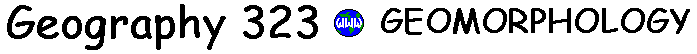# Slopes

• Mass wasting dominates the geomorphology of steep slopes in most environments (i.e. subhumid to humid)
• In dry environments (badlands) slopes are subject to episodic fluvial erosion

## Slope forms

### characteristic slopes

the angle of the dominant slope segment that is achieved when the slope profile is in equilibrium with the predominant slope
• convex slope segments
• form on the upper parts of slopes in response to soil creep and rainsplash erosion, when slopes are below the threshold for rapid mass wasting
• concave slope segments
• depositional (e.g. talus) or transporational (e.g. pediments) slope segments that form near the base of slopes and in the absence of removal of waste (e.g. river downcutting)
• with increasing runoff downslope, velocity and sediment transport can be maintained over increasingly lower slopes
• straight slope segments
• mid-slope segments dominated by transfer of debris or removal at a uniform rate (e.g. shallow slides)
• also the lower parts of slopes where debris is removed from the base
• composite slopes
• most hillslopes consist of a series of segments, for example, convex-concave slopes with soil and vegetation: a convex upper segment, straight mid slope and basal concavity

### rock slopes

slope angles are controlled by geologic structure or rock mass strength, i.e. the resistance of rock units to gravitational and fluid stresses
• strength equilibrium slopes
• rock slope profiles that reflect the strength of the rock units that underlay the slope
• occur in massive or horizontally bedded rocks
• structurally-controled slopes
• in dipping or folded rocks where slope angles conform to geologic structures

## Slope evolution

• decline
• progressive decrease in slope angle as upper convexities and basal concavities from by creep, splash and wash and consume the straight segment
• replacement
• the straight segment retreats and is replaced by a lower wash slope
• parallel retreat
• uniform intensity if slope processes and/or constant rock strength and removal of debris from the base
[ Course Outline | Next Topic ]